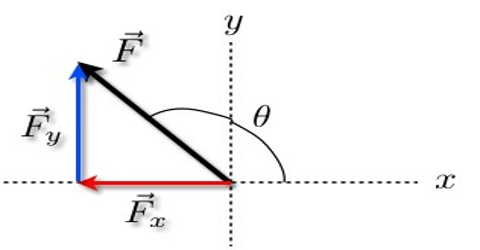# Vector Representation

Vector Representation

Different vector quantities are mathematically expressed in vector form. As a result, the magnitude and direction of the quantity become clear. Vector forms of some physical quantities are shown below:

Force: The external cause which changes or tends to change the state of rest or motion of a body is called force.

Force is a vector quantity. It has both direction and magnitude. If the mass of a moving body is “m”, its acceleration is ā and force acting on this body is F, then the vector representation is:

F = māVectors can be graphically represented by directed line segments. The length is preferred, according to some scale, to represent the magnitude of the vector, and the direction of the directed line segment represents the direction of the vector.